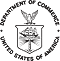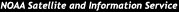formerly the National Oceanographic Data Center (NODC)...  more on NCEIThis Site All of NOAA
 You are here: NODC Home > Satellite Oceanography Team > GHRSST LTSRF > Transfer LogsMonth 2006 2007 2008 2009 2010 2011 2012 2013 2014 2015 2016 2017 2018
January x x x x x x x x x x x x x
February x x x x x x x x x x x x x
March x x x x x x x x x x x x x
April x x x x x x x x x x x x x
May x x x x x x x x x x x x x
June x x x x x x x x x x x x x
July x x x x x x x x x x x x x
August x x x x x x x x x x x x x
September x x x x x x x x x x x x x
October x x x x x x x x x x x x x
November x x x x x x x x x x x x x
December x x x x x x x x x x x x x
Year Summary x x x x x x x x x x x x x

Month 2006 2007 2008 2009 2010 2011 2012 2013 2014 2015 2016 2017 2018
January x x x x x x x x x x x x x
February x x x x x x x x x x x x x
March x x x x x x x x x x x x x
April x x x x x x x x x x x x x
May x x x x x x x x x x x x x
June x x x x x x x x x x x x x
July x x x x x x x x x x x x x
August x x x x x x x x x x x x x
September x x x x x x x x x x x x x
October x x x x x x x x x x x x x
November x x x x x x x x x x x x x
December x x x x x x x x x x x x x
Year Summary x x x x x x x x x x x x x

Month 2006 2007 2008 2009 2010 2011 2012 2013 2014 2015 2016 2017 2018
January x x x x x x x x x x x x x
February x x x x x x x x x x x x x
March x x x x x x x x x x x x x
April x x x x x x x x x x x x x
May x x x x x x x x x x x x x
June x x x x x x x x x x x x x
July x x x x x x x x x x x x x
August x x x x x x x x x x x x x
September x x x x x x x x x x x x x
October x x x x x x x x x x x x x
November x x x x x x x x x x x x x
December x x x x x x x x x x x x x
Year Summary x x x x x x x x x x x x xNODC NOAA CLASS AVHRR SST GODAE GAC RSMAS GHRSST GHRSST-PP MCSST NLSST SeaWiFS OAIS AIP SIP DIP
GOSTA NPOESS VIIRS OPeNDAP LAS HRPT LAC GAC HDF-SDS DMAC PO.DAAC LTSRF GDAC RDAC L2P L4 OSTIA

 Last modified:    Thu, 5-Sep-2013 22:57 UTC NCEI.info@noaa.gov Dept. of Commerce - NOAA - NESDIS - NCEI * Offsite Link Notification Like us on Facebook USA.gov - The U.S. Government's Web Portal